Get inspired by the success stories of our students in IIT JAM MS, ISI  MStat, CMI MSc DS.  Learn More

# ISI MStat PSB 2015 Question 8 | MLE & amp | Stochastic RegressionThis is a problem involving BLUE for regression coefficients and MLE of a regression coefficient for a particular case of the regressors. This problem is from ISI MStat PSB 2015 Question 8.

## The Problem- ISI MStat PSB 2015 Question 8

Consider the regression model: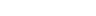,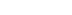where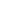are fixed non-zero real numbers and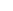's are independent random variables with mean 0 and equal variance.

(a) Consider estimators of the form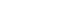(where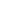's are non random real numbers) that are unbiased for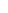. Show that the least squares estimator ofhas the minimum variance in this class of estimators.

(b) Suppose that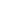's take values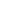or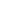and's have density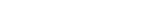.

Find the maximum likelihood estimator of.

## Pre-requisites:

1.Linear estimation

2.Minimum Variance Unbiased Estimation

3.Principle of Least Squares

4.Finding MLE

## Solution:

Clearly, part(a) is a well known result that the least squares estimator is the BLUE(Best linear unbiased estimator) for the regression coefficients.

You can probably look up its proof in the internet or in any standard text on linear regression.

Here's take values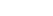. But the approach still remains the same.

Let's look at the likelihood function of: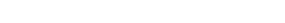or,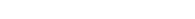where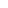is an appropriate constant (unimportant here)

Maximizing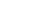w.r.tis same as minimizing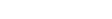w.r.t ..

Note that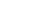. Let us define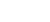.

Here's the catch now: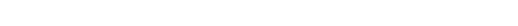.

Now remember your days when you took your first baby steps in statistics , can you remember the result that "Mean deviation about median is the least" ?

So,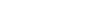is minimized for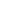Median.

Thus, MLE ofis the median of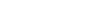.

## Food For Thought:

In classical regression models we assume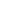's are non-stochastic. But is it really valid always? Not at all.

In case of stochastic's , there is a separate branch of regression called Stochastic Regression, which deals with a slightly different analysis and estimates.

I urge the interested readers to go through this topic from any book/ paper .

You may refer Montgomery, Draper & Smith etc.

## Previous MStat Posts:

This is a problem involving BLUE for regression coefficients and MLE of a regression coefficient for a particular case of the regressors. This problem is from ISI MStat PSB 2015 Question 8.

## The Problem- ISI MStat PSB 2015 Question 8

Consider the regression model:,whereare fixed non-zero real numbers and's are independent random variables with mean 0 and equal variance.

(a) Consider estimators of the form(where's are non random real numbers) that are unbiased for. Show that the least squares estimator ofhas the minimum variance in this class of estimators.

(b) Suppose that's take valuesorand's have density.

Find the maximum likelihood estimator of.

## Pre-requisites:

1.Linear estimation

2.Minimum Variance Unbiased Estimation

3.Principle of Least Squares

4.Finding MLE

## Solution:

Clearly, part(a) is a well known result that the least squares estimator is the BLUE(Best linear unbiased estimator) for the regression coefficients.

You can probably look up its proof in the internet or in any standard text on linear regression.

Here's take values. But the approach still remains the same.

Let's look at the likelihood function of:or,whereis an appropriate constant (unimportant here)

Maximizingw.r.tis same as minimizingw.r.t ..

Note that. Let us define.

Here's the catch now:.

Now remember your days when you took your first baby steps in statistics , can you remember the result that "Mean deviation about median is the least" ?

So,is minimized forMedian.

Thus, MLE ofis the median of.

## Food For Thought:

In classical regression models we assume's are non-stochastic. But is it really valid always? Not at all.

In case of stochastic's , there is a separate branch of regression called Stochastic Regression, which deals with a slightly different analysis and estimates.

I urge the interested readers to go through this topic from any book/ paper .

You may refer Montgomery, Draper & Smith etc.

## Previous MStat Posts:

This site uses Akismet to reduce spam. Learn how your comment data is processed.

### Knowledge Partner